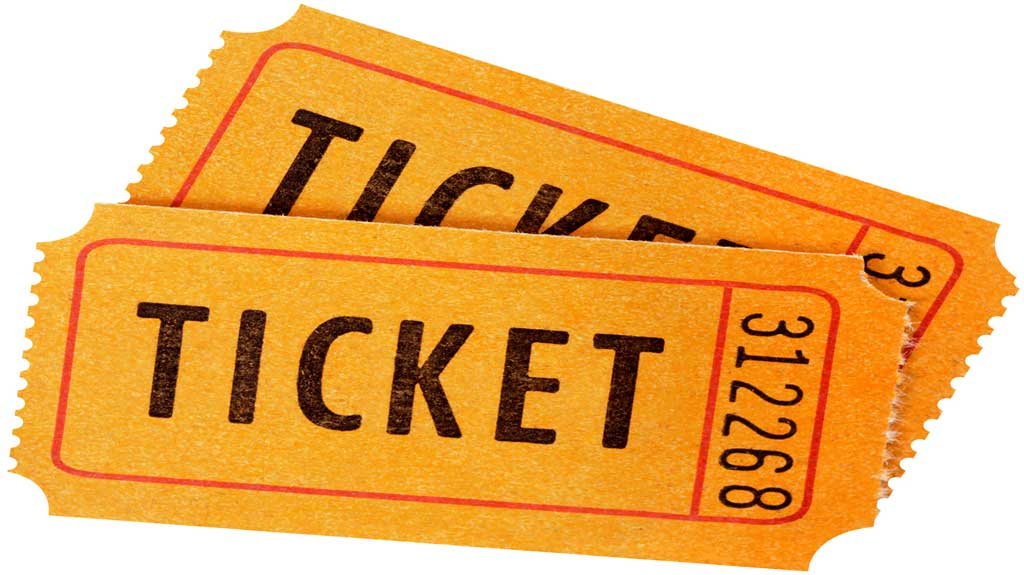Sunday, April 18, 2021# Algebra 1 PARCC question: build function

-

#### The following multi-part (constructed response and fill-in-the-blank) question, explained here in hopes of helping algebra students in Maryland and Illinois prepare for the PARCC test near the end of this school year, appears on the released version of PARCC’s Spring 2015 test in algebra 1, here:A school is holding a raffle to earn money. The list shows all the prizes in the school’s raffle.

• A computer that costs $349 • A book collection that costs$42
• A gift certificate that costs $25 • A pair of movie tickets that costs$18
• A gift basket that costs $16 The raffle ticket price is set so that 75 raffle tickets will pay for all of the prizes. Part A Create a function that can be used to find the total amount of money the school earns by selling x tickets. Show your work used to create this function. Enter your function and your work in the space provided. Part B The school’s goal is to raise at least$850 more than the total cost of the prizes. What is the minimum number of raffle tickets that have to be sold in order for the school to reach its goal?

Enter your answer in the box.

____ tickets

Answer and references

Correct answers: A: f(x) = 6x – 450 (work is human-scored). B: 217 (machine-scored).

PARCC evidence statement(s) tested: HS.D.2-9:

Solve multi-step contextual word problems with degree of difficulty appropriate to the course, requiring application of course-level knowledge and skills articulated in F-BF.1a, F-BF.3, A-CED.1, A-SSE.3, F-IF.B, F-IF.7, limited to linear and quadratic functions.

F-BF.1a (write a function that describes a relationship between two quantities; determine an explicit expression, a recursive process, or steps for calculation from a context) is the primary content; other listed content elements may be involved in tasks as well.

The evidence statement above references Math Practice 2 and Math Practice 4 in the Common Core:

[MP2] Reason abstractly and quantitatively. Mathematically proficient students make sense of quantities and their relationships in problem situations. They bring two complementary abilities to bear on problems involving quantitative relationships: the ability to decontextualize—to abstract a given situation and represent it symbolically and manipulate the representing symbols as if they have a life of their own, without necessarily attending to their referents—and the ability to contextualize, to pause as needed during the manipulation process in order to probe into the referents for the symbols involved. Quantitative reasoning entails habits of creating a coherent representation of the problem at hand; considering the units involved; attending to the meaning of quantities, not just how to compute them; and knowing and flexibly using different properties of operations and objects.

[MP4] Model with mathematics. Mathematically proficient students can apply the mathematics they know to solve problems arising in everyday life, society, and the workplace. In early grades, this might be as simple as writing an addition equation to describe a situation. … By high school, a student might use geometry to solve a design problem or use a function to describe how one quantity of interest depends on another. Mathematically proficient students … are able to identify important quantities in a practical situation and map their relationships using such tools as diagrams, two-way tables, graphs, flowcharts and formulas. They can analyze those relationships mathematically to draw conclusions. They routinely interpret their mathematical results in the context of the situation and reflect on whether the results make sense, possibly improving the model if it has not served its purpose.

The question tests students’ understanding of the high school Common Core standard HSF.BF.A.1, found under high school functions (building functions), which states that they should be able to “write a function that describes a relationship between two quantities” and [BF.A.1.A] “determine an explicit expression, a recursive process, or steps for calculation from a context.”

Example of a solution strategy (there are others)

Model the scenario that selling 75 tickets at x dollars will pay for all the prizes.

The prizes in total cost $450 (349 + 42 + 25 + 18 + 16). The price is set so that 75 tickets will make that much money.$\frac{450}{75} = 6$ So, the ticket price is$6 and the school will earn 6x dollars by selling x tickets, but the total cost of the prizes will have to be subtracted from that. Therefore, when the school sells x tickets, the function that defines the amount the school will earn is as follows:$f(x) = 6x - 450$

That’s Part A. The Part B of the problem wants to know just a number, and no explanation is required to receive full credit. However, let me explain a little.

If the school wants to earn $850 more than$450, the question comes down to how many tickets does the school need to sell at $6 apiece to get$1300?$6x = 1300$$\frac{6x}{6} = \frac{1300}{6}$$x = 216\frac{2}{3}$

## Purpose of this series of posts

Voxitatis is developing blog posts that address every algebra 1 question released to the public by the Partnership for Assessment of Readiness for College and Careers, or PARCC, in order to help students prepare to take the test this spring.

Our total release will run from February 27 through March 15, with one or two questions discussed per day. Then we’ll move to geometry at the end of March, algebra 2 during the first half of April, and eighth grade during the last half of April.Paul Katulahttps://news.schoolsdo.org
Paul Katula is the executive editor of the Voxitatis Research Foundation, which publishes this blog. For more information, see the About page.

### Md. to administer tests in math, English

0
Students in Md. will still have to take standardized tests this spring in math and English language arts, following action of the state board.